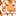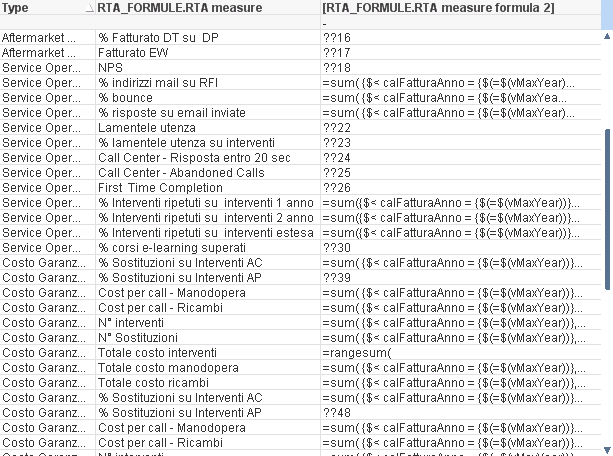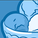# QlikView App Dev

Discussion Board for collaboration related to QlikView App Development.

Announcements
QlikWorld 2023, a live, in-person thrill ride. Save \$300 before February 6: REGISTER NOW!
cancel
Showing results for
Did you mean:Partner - Creator III

## Interpret field values as formulas

Hi there, is there a way to interpret the content of a field as a formula and execute it? I have a table whose third field contains formula expressions and I want to execute instead of simply showing them.

Look at the picture below.I tried with \$ expansion and fieldvalue but it does not work.

1 Solution

Accepted SolutionsPartner - Creator III
Author

I found the solution (it's pretty similar to the one suggested in the post mentioned by Piet Hein van del Stigchel.

First of all, formulas must not start with "=" symbol; then, the pivot table expression can be set like this:

 pick([RTA_FORMULE.RTA measure index], \$(=fieldvalue('RTA_FORMULE.RTA measure formula 2',1)), \$(=fieldvalue('RTA_FORMULE.RTA measure formula 2',2)), \$(=fieldvalue('RTA_FORMULE.RTA measure formula 2',3)), \$(=fieldvalue('RTA_FORMULE.RTA measure formula 2',4)), \$(=fieldvalue('RTA_FORMULE.RTA measure formula 2',5)), ... )

Thanks

5 RepliesPartner - Master

\$ expansion in an expression is only evaluated once for the whole chart, so this will only work when there is 1 valid formula. Take a look at the following thread, maybe it can help you.

Using formulas from excel in the straight tablePartner - Creator III
Author

I found the solution (it's pretty similar to the one suggested in the post mentioned by Piet Hein van del Stigchel.

First of all, formulas must not start with "=" symbol; then, the pivot table expression can be set like this:

 pick([RTA_FORMULE.RTA measure index], \$(=fieldvalue('RTA_FORMULE.RTA measure formula 2',1)), \$(=fieldvalue('RTA_FORMULE.RTA measure formula 2',2)), \$(=fieldvalue('RTA_FORMULE.RTA measure formula 2',3)), \$(=fieldvalue('RTA_FORMULE.RTA measure formula 2',4)), \$(=fieldvalue('RTA_FORMULE.RTA measure formula 2',5)), ... )

ThanksCreator

\$ expansion in an expression is only evaluated once for the whole chart, so this will only work when there is 1 valid formula.Partner - Creator II

Hi Alberto, Could you please explain your solution in details, I have just tested it and seems I can not make it working!

I have similar fields in my db as your  [RTA_FORMULE.RTA measure index]  and [RTA_FORMULE.RTA measure formula 2]  where:

[RTA_FORMULE.RTA measure index]  is the row no

[RTA_FORMULE.RTA measure formula 2] is the formula

Thanks in advance! If possible could you upload a simple example! TnxContributor III

Ivan, did you ever find an example file using this? Thanks in advance.Community Browser